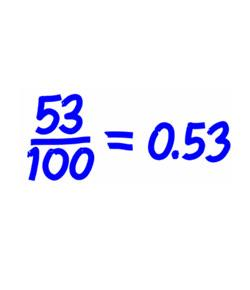# Year 5 Decimal Challenge!

4 Questions | Total Attempts: 1913SettingsCan you complete the decimal challenge? Test your knowledge against the quiz!

• 1.
How many tenths make a whole unit?
• A.

1

• B.

5

• C.

10

• D.

100

• 2.
What is 50% as a decimal fraction?
• A.

0.1

• B.

0.5

• C.

0.05

• D.

0.01

• 3.
What does   0.7 - 0.1 =  ?
• A.

0.8

• B.

0.07

• C.

0.06

• D.

0.6

• 4.
Add these two decimal fractions together, what is the answer?0.05 + 0.3 =
• A.

0.8

• B.

0.53

• C.

0.35

• D.

0.2

Related Topics